# How to Create a Residual Plot in Python

• Last Updated : 21 Feb, 2022

A residual plot is a graph in which the residuals are displayed on the y axis and the independent variable is displayed on the x-axis. A linear regression model is appropriate for the data if the dots in a residual plot are randomly distributed across the horizontal axis. Let’s see how to create a residual plot in python.

## Method 1: Using the plot_regress_exog()

plot_regress_exog():

• Compare the regression findings to one regressor.
• ‘endog vs exog,”residuals versus exog,’ ‘fitted versus exog,’ and ‘fitted plus residual versus exog’ are plotted in a 2 by 2 figure.

Syntax: statsmodels.graphics.regressionplots.plot_regress_exog(results, exog_idx, fig=None)

Parameters:

• results: result instance
• exog_idx: index or name of the regressor
• fig : a figure is created if no figure is provided

Returns: 2X2 figure

### Single Linear Regression

After importing the necessary packages and reading the CSV file, we use ols() from statsmodels.formula.api to fit the data to linear regression. we create a figure and pass that figure, name of the independent variable, and regression model to plot_regress_exog() method. a 2X2 figure of residual plots is displayed. In the ols() method the string before ‘~’ is the dependent variable or the variable which we are trying to predict and after ‘~’ comes the independent variables. for linear regression, there’s one dependent variable and one independent variable.

ols(‘response_variable ~ predictor_variable’, data= data)

## Python3

 `# import packages and libraries ` `import` `numpy as np ` `import` `pandas as pd ` `import` `matplotlib.pyplot as plt ` `import` `statsmodels.api as sm ` `from` `statsmodels.formula.api ``import` `ols ` ` `  `# reading the csv file ` `data ``=` `pd.read_csv(``'headbrain3.csv'``) ` ` `  `# fit simple linear regression model ` `linear_model ``=` `ols(``'Brain_weight ~ Head_size'``, ` `                   ``data``=``data).fit() ` ` `  `# display model summary ` `print``(linear_model.summary()) ` ` `  `# modify figure size ` `fig ``=` `plt.figure(figsize``=``(``14``, ``8``)) ` ` `  `# creating regression plots ` `fig ``=` `sm.graphics.plot_regress_exog(linear_model, ` `                                    ``'Head_size'``, ` `                                    ``fig``=``fig) `

Output:

We can see that the points are plotted randomly spread or scattered. points or residuals are scattered around the ‘0’ line, there is no pattern, and points are not based on one side so there’s no problem of heteroscedasticity.  with the predictor variable ‘Head_size’ there’s no heteroscedasticity.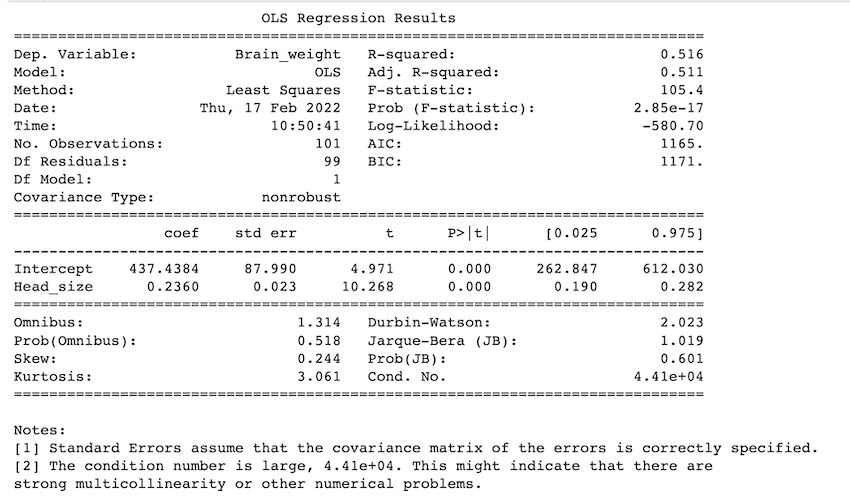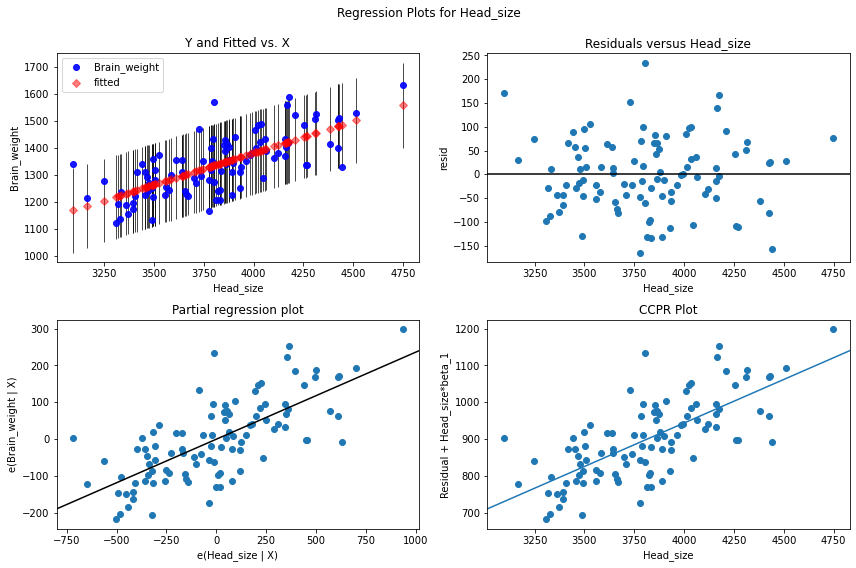### Multiple linear regression:

In multiple linear regression, we have more than independent variables or predictor variables and one dependent variable. The code is similar to linear regression except that we have to make this change in the ols() method.

ols(‘response_variable ~ predictor_variable1+ predictor_variable2 +…. ‘, data= data)

‘+’ is used to add how many ever predictor_variables we want while creating the model.

CSV Used: homeprices

Example 1:

## Python3

 `# import packages and libraries ` `import` `numpy as np ` `import` `pandas as pd ` `import` `matplotlib.pyplot as plt ` `import` `statsmodels.api as sm ` `from` `statsmodels.formula.api ``import` `ols ` ` `  `# reading the csv file ` `data ``=` `pd.read_csv(``'homeprices.csv'``) ` `data ` ` `  `# fit multi linear regression model ` `multi_model ``=` `ols(``'price ~ area + bedrooms'``, data``=``data).fit() ` ` `  `# display model summary ` `print``(multi_model.summary()) ` ` `  `# modify figure size ` `fig ``=` `plt.figure(figsize``=``(``14``, ``8``)) ` ` `  `# creating regression plots ` `fig ``=` `sm.graphics.plot_regress_exog(multi_model, ``'area'``, fig``=``fig) `

Output:

We can see that the points are plotted randomly spread or scattered. points or residuals are scattered around the ‘0’ line, there is no pattern, and points are not based on one side so there’s no problem of heteroscedasticity.  With the predictor variable ‘area’ there’s no heteroscedasticity.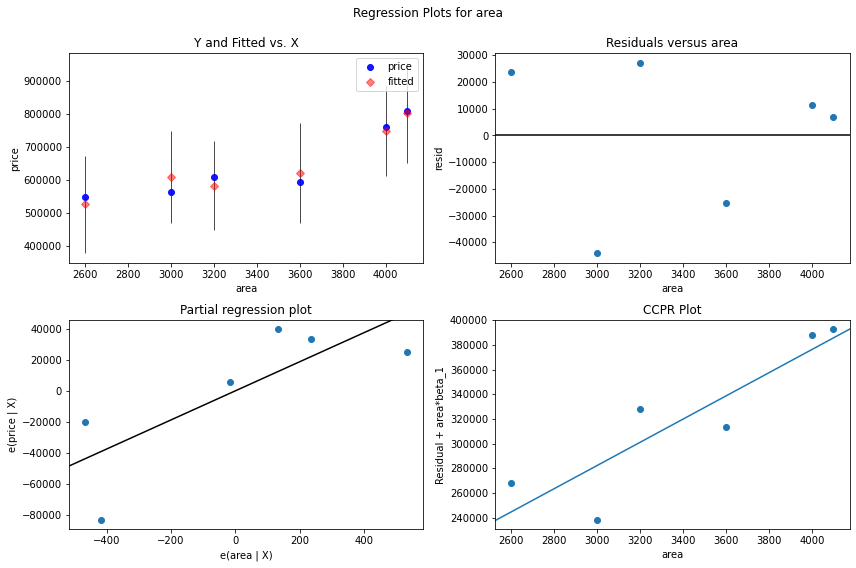Example 2:

## Python3

 `# import packages and libraries ` `import` `numpy as np ` `import` `pandas as pd ` `import` `matplotlib.pyplot as plt ` `import` `statsmodels.api as sm ` `from` `statsmodels.formula.api ``import` `ols ` ` `  `# reading the csv file ` `data ``=` `pd.read_csv(``'homeprices.csv'``) ` `data ` ` `  `# fit multi linear regression model ` `multi_model ``=` `ols(``'price ~ area + bedrooms'``, data``=``data).fit() ` ` `  `# modify figure size ` `fig ``=` `plt.figure(figsize``=``(``14``, ``8``)) ` ` `  `# creating regression plots ` `fig ``=` `sm.graphics.plot_regress_exog(multi_model, ``'bedrooms'``, fig``=``fig) `

Output:

we can see that the points are plotted randomly spread or scattered. points or residuals are scattered around the ‘0’ line, there is no pattern and points are not based on one side so there’s no problem of heteroscedasticity.  with the predictor variable ‘bedrooms’ there’s no heteroscedasticity.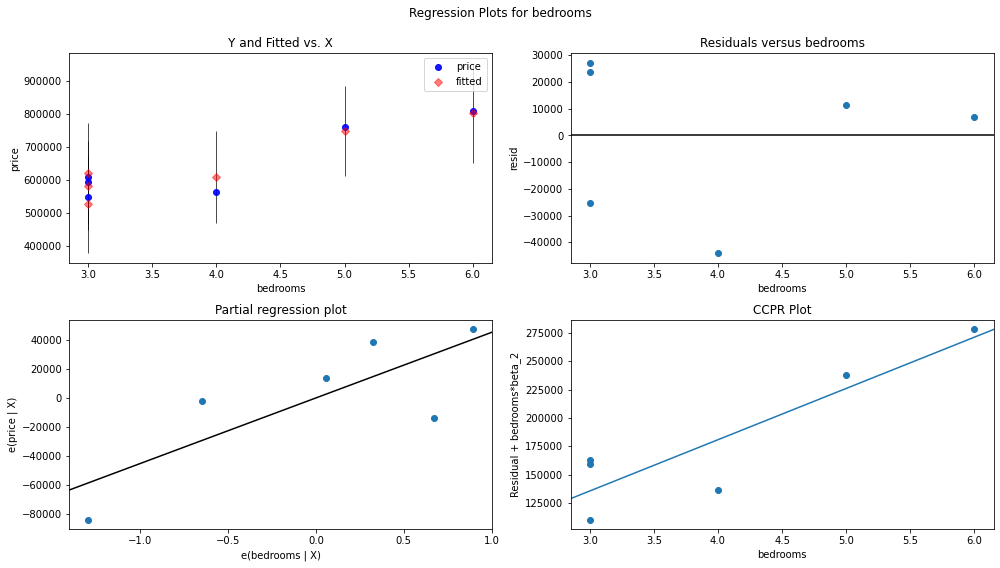## Method 2: Using seaborn.residplot()

seaborn.residplot(): This function will regress y on x  and then plot the residuals as a scatterplot. You can fit a lowess smoother to the residual plot as an option, which can aid in detecting whether the residuals have structure.

Syntax: seaborn.residplot(*, x=None, y=None, data=None, lowess=False, x_partial=None, y_partial=None, order=1, robust=False, dropna=True, label=None, color=None, scatter_kws=None, line_kws=None, ax=None)

Parameters:

• x : column name of the independent variable (predictor) or a vector.
• y: column name of the dependent variable(response) or a vector.
• data: optional parameter. dataframe
• lowess: by default it’s false.

Below is an example of a simple residual plot where x(independent variable) is head_size from the dataset and y(dependent variable) is the brain_weight column of the dataset.

## Python3

 `# import packages and libraries ` `import` `pandas as pd ` `import` `seaborn as sns ` `import` `matplotlib.pyplot as plt ` ` `  `# reading the csv file ` `data ``=` `pd.read_csv(``'headbrain3.csv'``) ` ` `  `sns.residplot(x``=``'Head_size'``, y``=``'Brain_weight'``, data``=``data) ` ` `  `plt.show() `

Output:

We can see that the points are plotted in a randomly spread, there is no pattern and points are not based on one side so there’s no problem of heteroscedasticity.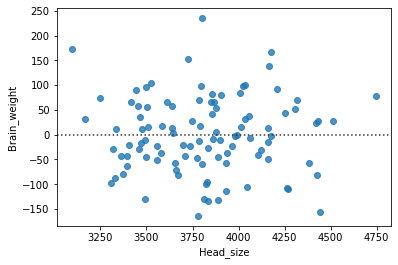My Personal Notes arrow_drop_up
Recommended Articles
Page :